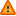FAQ/wavelength_eg - CBU statistics WikiYou should trust both wikis because the password could be read by the particular administrators.

Clear message
location: FAQ / wavelength_eg

# Working out 2 group signal detection diagnostics

The area under Receiver Operating Characteristic (ROC) curves is a way of describing the magnitude of how well separated two groups are with respect to some diagnostic.

For two groups where the mean of A > mean of B it is a plot of sensitivity at each of T thresholds (Probability of being in group A given you have a value less than or equal to t) against 1 - specificity (probability of being in group B given you have a value less than or equal to t) as seen here.

The sensitivity is the blue horizontal area and 1-specificity is the diagonal orange area evaluated at a score of 1. These can also be evaulated using frequencies. You can see that as sensitivity increases so does 1-specificity (with the threshold increasing ie line moving to the right). When the line is at the far left both sensitivity and 1-specificity are zero and when the line is on the far right they are both equal to unity.

 True A B Score <= t a b > t c d

In the above table the sensitivity is the proportion of observations in group A which have values less than or equal to t and 1-specificity is the proportion of observations in group B which have a value less than or equal to a particular score t.

These probabilities are evaluated at each observed score, t and plotted with sensitivity on the y-axis and 1-specificity on the x-axis. The points may be joined together to form a curve and the area under the curve evaluated using, for example, the trapezium rule. The area under the ROC curve may be computed on the raw data using the LOGISTIC REGRESSION procedure in SPSS or a SPSS macro.